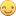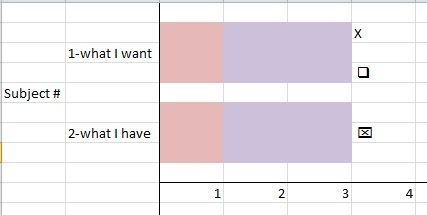## SGPLOT swimlane with different symbols at same timepoint

Asking for a co-worker (no, really I am!)so I don't have specific code (I'm not on his project...)

He is making a swimlane plot with subject ID on the y-axis and timepoint on the x-axis.  There are only a few subjects, so the bars are "thick" to fill up some space.

On these bars he is placing 2 symbols--X and a square--representing events that are not necessarily related, using scatterplot.  However, we have subjects for whom these 2 events happened at the same timepoint:Subject #2 shows how these symbols actually print--the two of them overlay each other.  However, the client wants it displayed like subject #1, with the symbols stacked.

Any ideas?

Many thanks!

VCM

2 REPLIES 2Jay54
Meteorite | Level 14

## Re: SGPLOT swimlane with different symbols at same timepoint

Try using the JITTER option on the SCATTER statement.Ksharp
Super User

## Re: SGPLOT swimlane with different symbols at same timepoint

``````data x;
x='x';y=1;a=1.2;b=1.2;
run;

proc sgplot data=x;
vbarparm category=x response=y  ;
scatter x=x y=a/ discreteoffset=-0.4 markerattrs=(symbol=X size=14);
scatter x=x y=b/ discreteoffset=0.4 markerattrs=(symbol=square size=14);
run;``````
Discussion stats
• 2 replies
• 794 views
• 0 likes
• 3 in conversation